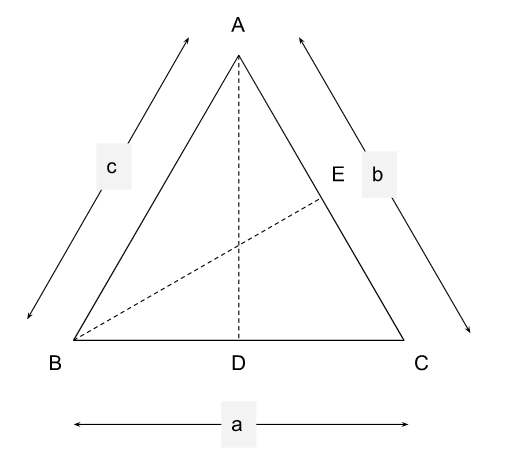# CAT 2022 Question Paper | Quant Slot 2

###### CAT Previous Year Paper | CAT Quant Questions | Question 1

CAT 2022 Quant was dominated by Arithmetic followed by Algebra. In Arithmetic, the questions were dominated by topics like Speed-time-distance, Mixture and Alligations. This year, there was a surprise. The questions from Geometry were relatively on the lower side as compared to the previous years. There were 8 TITA Qs this year. Overall this section was at a medium level of difficulty.

Question 1 : In triangle $A B C$, altitudes $A D$ and $B E$ are drawn to the corresponding bases. If $$angle B A C=45^{$circ}$ and $\angle A B C=\theta$, then $\frac{A D}{B E}$ equals 1. $\sqrt{2} \sin \theta$ 2. $\sqrt{2} \cos \theta$ 3. $\frac{$$sin \theta+\cos \theta$}{$sqrt{2}}$ 4. 1 ## Best CAT Online Coaching Try upto 40 hours for free Learn from the best! #### 2IIM : Best Online CAT Coaching. ### Video Explanation ## Best CAT Coaching in Chennai #### CAT Coaching in Chennai - CAT 2022Limited Seats Available - Register Now! ### Explanatory AnswerLet ‘a’, ‘b’ and ‘c’ be the length of the sides opposite to the vertices A, B and C respectively. Let the area of the triangle be Δ. By the sine rule, Δ = $\frac { 1 } { 2 } \times a \times c \times \sin$ B$$
Δ = $$frac { 1 } { 2 } $times b \times c \times \sin$ A$$
This implies,
$$frac { a } { b } = $frac { \sin$ B$ } { $sin$ A ) } = $frac { $sin$ $theta$ } { $sin$ 45$ } = $sqrt { 2 } $sin$ $theta$$ Area of the triangle, Δ is also given by, Δ = $$frac { 1 } { 2 } \times a \times A D$ Δ = $\frac { 1 } { 2 } \times b \times B E$ This implies, $\frac { a } { b } = \frac { A D } { B E }$ $\frac { a } { b } = \frac { A D } { B E } = \sqrt { 2 } \sin$ $theta$$ $$frac { A D } { B E } = \sqrt { 2 } \sin$ $theta$$ The question is " In triangle $A B C$, altitudes $A D$ and $B E$ are drawn to the corresponding bases. If $$angle B A C=45^{\circ}$ and $\angle A B C=\theta$, then $\frac{A D}{B E}$ equals " ##### Hence, the answer is '$\sqrt{2} \sin \theta$' Choice A is the correct answer. ###### Prepare for CAT 2023 with 2IIM's Daily Preparation Schedule ###### Know all about CAT Exam Syllabus and what to expect in CAT ###### Best CAT Online Coaching Try upto 40 hours for free Learn from the best! ###### Already have an Account? ###### CAT Coaching in ChennaiCAT 2023 Classroom Batches Starting Now! @Gopalapuram ###### Best CAT Coaching in Chennai Introductory offer of 5000/- Attend a Demo Class ###### Best Indore IPM & Rohtak IPM CoachingSignup and sample 9 full classes for free. Register now! ##### Where is 2IIM located? 2IIM Online CAT Coaching A Fermat Education Initiative, 58/16, Indira Gandhi Street, Kaveri Rangan Nagar, Saligramam, Chennai 600 093 ##### How to reach 2IIM? Mobile:$91$ 99626 48484 / 94459 38484
WhatsApp: WhatsApp Now
Email: info@2iim.com